Addition uses the `+` symbol in Excel, and is also known as plus.

There are two ways to do addition in Excel. Either by using the `+` symbol in a formula or by using the SUM function.

1. Select a cell and type (`=`)
2. Select a cell
3. Type (`+`)
4. Select another cell
5. Hit enter

You can add more cells to the formula by typing (+) between the cells.

Let's have a look at some examples.

• Type `A1(=)`
• Type `5+5`
• Hit enter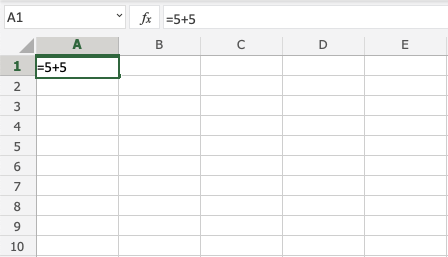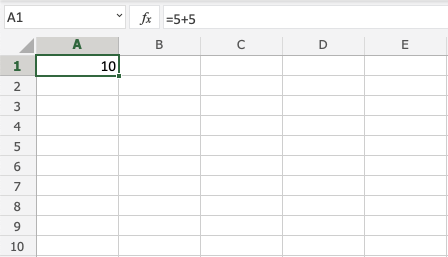Congratulations! You have successfully added `5+5=10`.

First let's add some numbers to work with. Type the following values: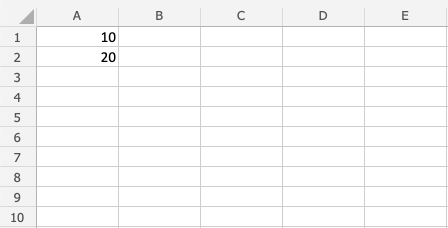How to do it, step by step:

1. Type `B1(=)`
2. Select `A1`
3. Type (`+`)
4. Select `A2`
5. Hit enter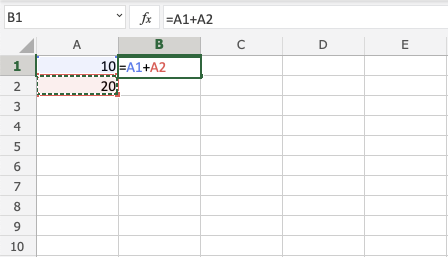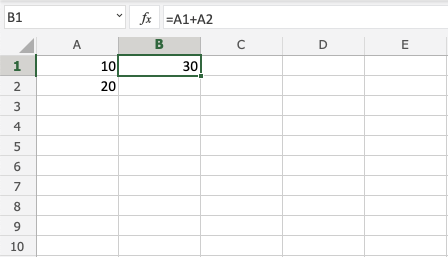Great! `30` is the result by adding `A1` and `A2`.

First let's add some numbers to work with. Type the following values: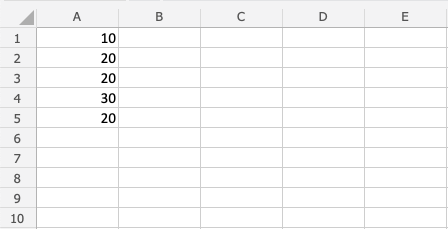Step by step to add several cells:

1. Type `B1(=)`
2. Select `A1`
3. Type (`+`)
4. Select `A2`
5. Type (`+`)
6. Select `A3`
7. Type (`+`)
8. Select `A4`
9. Type (`+`)
10. Select `A5`
11. Hit enter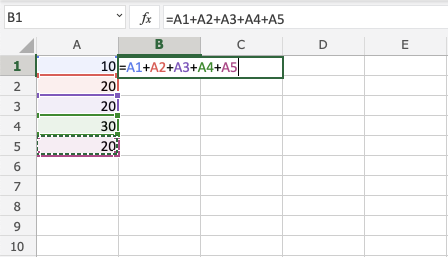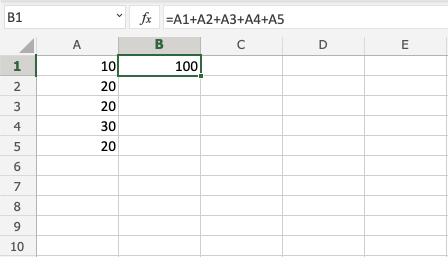Good job! You have successfully added five cells!

Let's keep the numbers from the last exercise. If you did last exercise, remove the value in `B1`.Step by step to add with SUM:

1. Type `B1(=SUM)`
2. Double click the `SUM` command
3. Mark the range `A1:A5`
4. Hit enter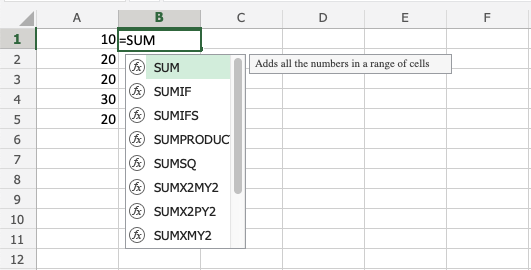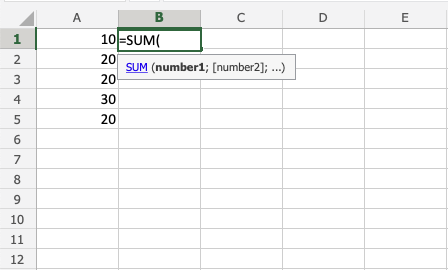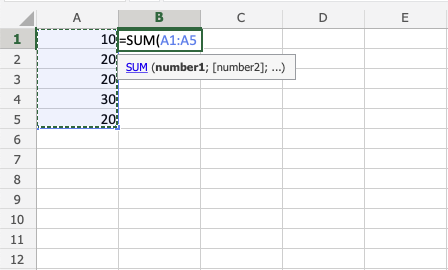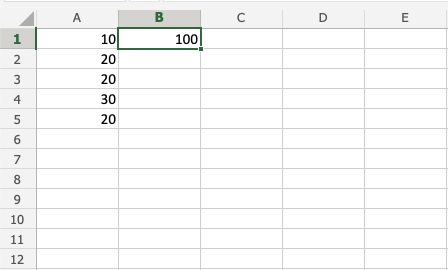Note: `SUM` saves you time! Keep practicing this function.

You can also lock a cell and add it to other cells.

How to do it, step by step:

1. Select a cell and type (`=`)
2. Select the cell you want to lock, add two dollar signs (`\$`) before the column and row
3. Type (`+`)
4. Fill a range

Let's have a look at an example where we add `B(5)` to the range `A1:A10` using absolute reference and the fill function.

Type the values: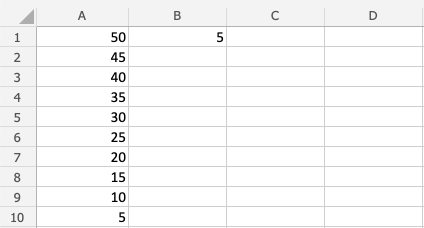Step by step:

1. Type `C1(=)`
2. Select `B1`
3. Type dollar sign before column and row `\$B\$1`
4. Type (`+`)
5. Select `A1`
6. Hit enter
7. Fill the range `C1:C10`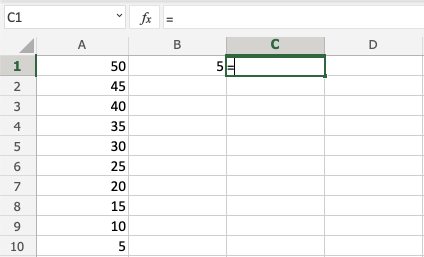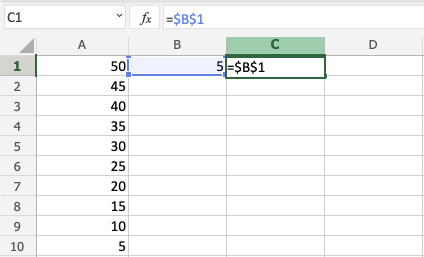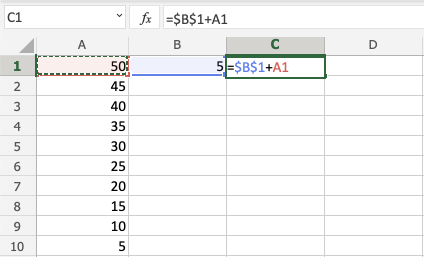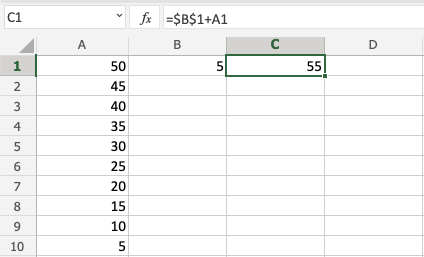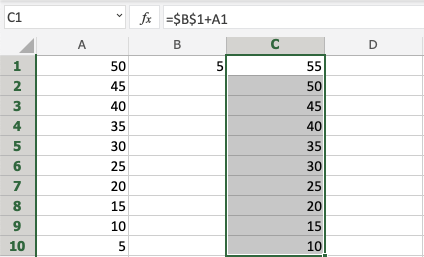Great! You have successfully used absolute reference to add `B1(5)` with the range `A1:A10`.

## Excel Exercise:

Type the symbol that Excel uses for addition:

```
```

Start the Exercise